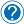Converting strings to numbers in javascript

There are some situations where you would want to take a string from a place, convert it to a number and put it in another place on the client side. This thing can be obtained by some helper function that are built-in in javascript.

In this article we will use these functions: parseInt(), parseFloat() and toString(). There is no problem if you didn’t use these in the past, I will explain them below in detail.

First, let’s see what are the possible data type conversions:

1) String to Integer (int)
2) Float to Integer (int)
3) String to Float
4) Integer (int) to String
5) Float to String

Converting String to Integer

To convert a value from string to integer we have to use the function “parseInt()”. This function parses a string and returns an integer.

Syntax:

Parameters:

@string = Required. The string to be parsed

@radix = optional. A number (from 2 to 36) that represents the numeral system to be used

Example:

 Example Result parseInt(“4”); 4 parseInt(“5axfa”); 5 parseInt(“4.333”); 4 parseInt(“asdas”); NaN (it means “Not a Number”)

Converting Float to Integer

To do this conversion we also use parseInt() function.

Example:

Converting String to Float

To convert  from string to float, we have to use another function called “parseFloat()” to convert a string value to float. Let’s see an working example with this:

Converting Integer/Float to String

Integer or float values can be converted to string by using the function or method “toString()”.

And that’s it really, pretty easy if I may add. What you do need to know though, javascript functions are case sensitive, meaning tostring() will not be the same as toString() so make sure you use the functions properly.

Until next time, cheers.Request an article ←